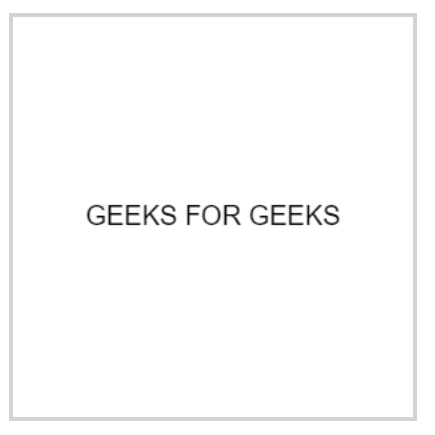Open in App
Not now

# How to sharpen blurry text in HTML5 canvas?

• Last Updated : 14 Aug, 2020

For getting a solution to this problem, let us first understand the problem that Why do this problem take place.

The answer to this Question is the Pixels of the screen. The amount of blur often depends on the browser or the device you are using to view the Canvas. The Pixel ratios vary for different devices and so we get to see blurry effects.

Solution to the Problem:

We can solve this problem by using window.devicepixelratio property

The devicePixelRatio of Window interface returns the ratio of the resolution in physical pixels to the resolution in CSS pixels for the current display device. This value could also be interpreted as the ratio of pixel sizes: the size of one CSS pixel to the size of one physical pixel.

In simpler terms, this tells the browser how many of the screen’s actual pixels should be used to draw a single CSS pixel.

Let’s see an example of how our output looks without using devicePixelRatio:Let’s now write some code to resolve this issue:

 ```<``html``>`` ` `<``body``>``    ``<``canvas` `id``=``"canvas"` `            ``style``=``"border:1px solid #d3d3d3;"``>``    ````    ``<``script``>``        ``var canvas = document.getElementById('canvas');``        ``var ctx = canvas.getContext('2d');``              ``//window.devicePixelRatio=1; //Blury Text``        ``window.devicePixelRatio=2;      //Clear Text``        ``//(CSS pixels).``              ``//Display Size``        ``var size = 150;``        ``canvas.style.width = size + "px";``        ``canvas.style.height = size + "px";`` ` `        ``var scale = window.devicePixelRatio; ``           ` `        ``canvas.width = Math.floor(size * scale);``        ``canvas.height = Math.floor(size * scale);`` ` `        ``//CSS pixels for coordinate systems``        ``ctx.scale(scale, scale);``        ``ctx.font = '10px Arial';``        ``ctx.textAlign = 'center';``        ``ctx.textBaseline = 'middle';`` ` `        ``var x = size / 2;``        ``var y = size / 2;`` ` `        ``var textString = "GEEKS FOR GEEKS";``        ``ctx.fillText(textString, x, y);``    ```` ` ````

Output:

After including the window.devicePixelRatio let’s check the output for our code:We have assumed both the conditions here, when we keep the window.devicePixelRatio=1 we get to see the blurry effect but when we keep the value as 2 we can see that we got cleartext. This is because a value of 1 indicates a classic 96 DPI (76 DPI on some platforms) display, while a value of 2 is expected for HiDPI/Retina displays.

My Personal Notes arrow_drop_up